# 10 Excel Formulas Every Beginner Should Know

Excel formulas make calculating numbers and making sense of large amounts of data simple. By knowing a few key formulas, you can do a variety of actions in Excel that will increase your productivity and decrease the risk of making calculation mistakes. We’ve put together 10 Excel formulas you need to get started.

There are a lot of complicated formulas out there, but a great formula doesn’t have to be complex. In fact, some of the simplest formulas are the most useful and will help you maximize the capabilities of Excel.

Here are 10 Excel formulas you must know, including some simple formulas that don’t have anything to with data analysis, in case you’re using Excel to organise information too.

## 1. 10 Excel Formulas =SUM

=SUM is a great basic formula to know, especially because it allows you to add up numbers in different ways. Excel easily performs this formula for you, but there are a few tricks to =SUM that provide even more functionality for adding data.

First, =SUM can add up entire rows of numbers or just certain cells within a row. Here is what that looks like:=SUM(A2:A9) adds up values in cells A2 through A9

=SUM(A2, A9) adds up values in cells A2 and A9

But you can take =SUM it one step further and combine it with other math functions too. In this example, =SUM (A2:A9)/5 adds up values in cells A2 through A9, then divides the sum by 5.

## 2. 10 Excel Formulas =MAX & =MIN

If you have a spreadsheet with a lot of numbers, this is a useful formula. With =MAX you can immediately find the largest number in your data set, and with =MIN you can find the smallest.

Use =MAX(SELECT CELLS:SELECT CELLS).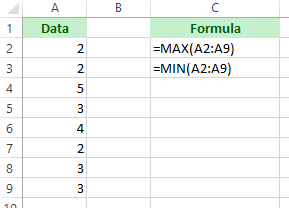=MAX(A2:A9)

=MIN (A2:A9)

## 3. 10 Excel Formulas =IF

With this formula, Excel will tell you if a certain condition is met. For example, you might need to know which values in column A are larger than 3. Using the =IF formula, you can get Excel to quickly auto-populate a “yes” for each cell that is larger than 3, and a “no” for each value that is smaller than 3. IF this one is not in your top 10 Excel formulas, you are missing out!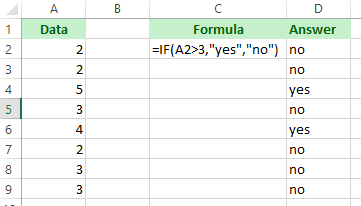=IF(A2>=3, “Yes”, “No”)

## 4. 10 Excel Formulas =TRIM

If you copy and paste data into a spreadsheet, there is a chance that the pasted data will be messy. That means it might have extra spaces or hidden characters, and these will mess up formulas because Excel needs data to be clean, without those extra spaces.

=TRIM cleans up pasted data so that it is Excel friendly.

In the example below, the animals in cells A3, A6, A8, A10 and A 12 have extra spaces at the beginning. Using =TRIM(SELECT A CELL) will fix it.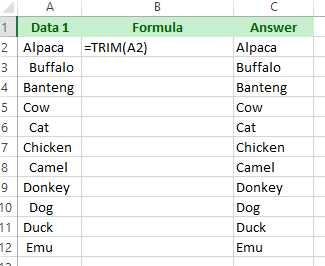You can see to the left how the formula has been used in Column D and the extra spaces have disappeared.

## 5. 10 Excel Formulas =CONCATENATE

=CONCATENATE is a useful formula that takes values from multiple cells and combines them into the same cell. This formula saves time and frustration when you need to combine the information in multiple cells into one cell. Instead of doing it manually, =CONCATENATE can do it, in half the time and half the clicks.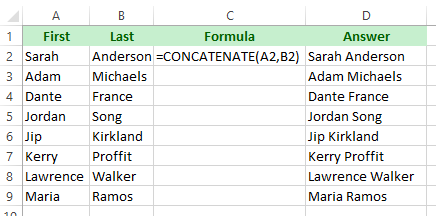In this example, Column A has first names and Column B has last names. Using =CONCATENATE(SELECT CELL, SELECT CELL) those cells can easily be combined, which is reflected in Column D, where the full names are now in the same cell. Think of all the copying/pasting this formula just saved!

## 6. 10 Excel Formulas =TODAY

You might find yourself needing to time stamp a spreadsheet each time it is viewed.Instead of typing in the date manually, use =TODAY(). That’s right – you don’t even have to put a value in the parenthesis, and each time the spreadsheet is opened it will update with the current date.

## 7. 10 Excel Formulas =PROPER

Excel isn’t just for data analysis; it is also a good platform for organizing and sorting information. When typing large amounts of text into Excel, =PROPER is a great formula to have in your pocket because it converts a cell of text to proper case, where the first letter of each word is capitalized, and the rest of the letters are lowercase.

In the example, quite a few names in Column A are not capitalized. Instead of clicking in each cell, deleting the first letter of each name and typing in a capitalised letter (which is a lot of extra clicks and time), =PROPER does it instantly, as you can see in Column D.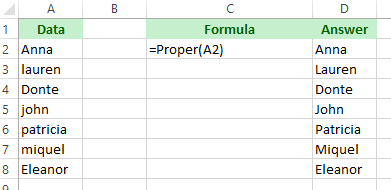Use =Proper(SELECT A CELL) for this formula.

## 8. 10 Excel Formulas =EVEN & =ODD

If you’re working with data that has a lot of decimals, this formula comes in handy. =EVEN rounds a number up to the nearest even number, and =ODD rounds a number up to the nearest odd number. If you’re working with negative numbers, these formulas still work, rounding down to the nearest even or odd number.In this example, Column D is using the =EVEN formula and column E is using the =ODD formula.

Use =EVEN(SELECT A CELL) and =ODD(SELECT A CELL) for this formula.

## 9. 10 Excel Formulas END OF MONTH

=EOMONTH can be used to find the last day of the current month or upcoming months. Instead of going back and forth between a calendar and the spreadsheet, use =EOMONTH(START DATE, 0). Take this formula a step further and calculate the next month by adding =EOMONTH(start-date, 1).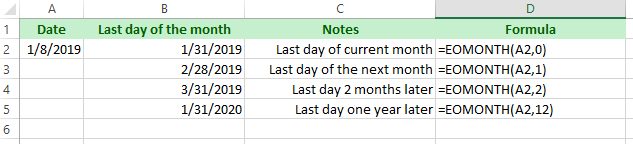Notice in the example how future months can be calculated by increasing the number at the end of the formula.

One more thing about this formula: when entering the start date, be sure to use the DATE function (2019,1,8) is January 8, 2019 so that the formula works currently. If the formula returns a #NUM! error, chances are that the date is not in the correct format.

## 10. 10 Excel Formulas RANDOM NUMBER GENERATOR

A spreadsheet can easily be used to select random numbers using =RANDBETWEEN(SELECT VALUES).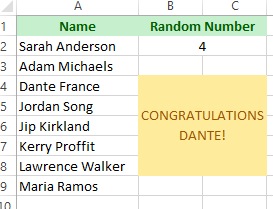Use this formula to choose numbers within data in the spreadsheet, as shown in the example to the left.

Another way to use this formula is to choose a winner from a list of 100 names, by having excel choose the winning row.

If you liked this article, then you’ll love our most popular course, Microsoft Excel. You’ll be an Excel expert in no time!